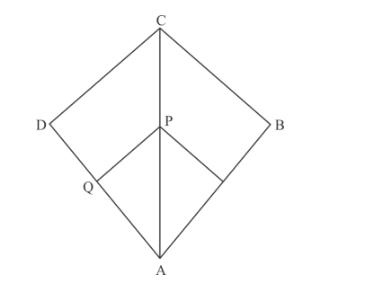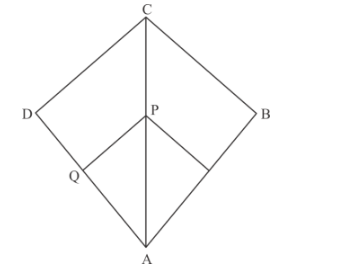# In Figure 3, PQ || CD and PR || CB. Prove that`
Question:

In Figure $3, P Q \| C D$ and $P R \| C B$. Prove that $\frac{A Q}{Q D}=\frac{A R}{R B}$.Solution:

Given that:

$P Q \| C D$ and $P R \| C B$, then we to prove that $\frac{A Q}{Q D}=\frac{A R}{R B}$

The following diagram is givenWe can easily see that, in the above figureare similar triangles, and also theare similar triangles.

Now, we have the following properties of similar triangles,

$\frac{A Q}{Q D}=\frac{A P}{P C}$     ................(1)

$\frac{A R}{R B}=\frac{A P}{P C}$      ................(2)

From equation (1) and Equation (2), we get

$\frac{A Q}{Q D}=\frac{A R}{R B}$

Hence proved.